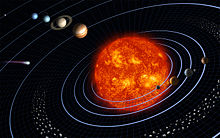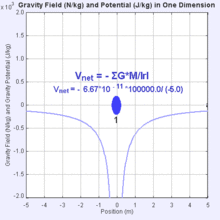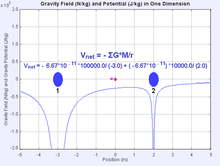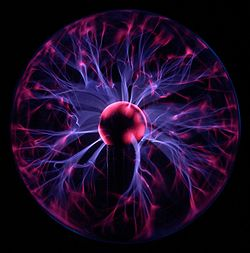# Potential energy

Potential energy

In physics, potential energy is the energy stored in a body or in a system due to its position in a force field or due to its configuration. The SI unit of measure for energy and work is the Joule (symbol J). The term "potential energy" was coined by the 19th century Scottish engineer and physicist William Rankine.

## Overview

Potential energy exists when a force acts upon an object that tends to restore it to a lower energy configuration. This force is often called a restoring force. For example, when a spring is stretched to the left, it exerts a force to the right so as to return to its original, unstretched position. Similarly, when a mass is lifted up, the force of gravity will act so as to bring it back down. The action of stretching the spring or lifting the mass requires energy to perform. The energy that went into lifting up the mass is stored in its position in the gravitational field, while similarly, the energy it took to stretch the spring is stored in the metal. According to the law of conservation of energy, energy cannot be created or destroyed; hence this energy cannot disappear. Instead, it is stored as potential energy. If the spring is released or the mass is dropped, this stored energy will be converted into kinetic energy by the restoring force, which is elasticity in the case of the spring, and gravity in the case of the mass. Think of a roller coaster. When the coaster climbs a hill it has potential energy. At the very top of the hill is its maximum potential energy. When the car speeds down the hill potential energy turns into kinetic. Kinetic energy is greatest at the bottom.

The more formal definition is that potential energy is the energy difference between the energy of an object in a given position and its energy at a reference position.

There are various types of potential energy, each associated with a particular type of force. More specifically, every conservative force gives rise to potential energy. For example, the work of an elastic force is called elastic potential energy; work of the gravitational force is called gravitational potential energy; work of the Coulomb force is called electric potential energy; work of the strong nuclear force or weak nuclear force acting on the baryon charge is called nuclear potential energy; work of intermolecular forces is called intermolecular potential energy. Chemical potential energy, such as the energy stored in fossil fuels, is the work of the Coulomb force during rearrangement of mutual positions of electrons and nuclei in atoms and molecules. Thermal energy usually has two components: the kinetic energy of random motions of particles and the potential energy of their mutual positions.

As a general rule, the work done by a conservative force F will be$\,W = -\Delta U$

where ΔU is the change in the potential energy associated with that particular force. Common notations for potential energy are U, V, Ep, and PE.

## Reference level

The potential energy is a function of the state a system is in, and is defined relative to that for a particular state. This reference state is not always a real state, it may also be a limit, such as with the distances between all bodies tending to infinity, provided that the energy involved in tending to that limit is finite, such as in the case of inverse-square law forces. Any arbitrary reference state could be used, therefore it can be chosen based on convenience.

Typically the potential energy of a system depends on the relative positions of its components only, so the reference state can also be expressed in terms of relative positions.

## Gravitational potential energy

Gravitational energy is the potential energy associated with gravitational force. If an object falls from one point to another point inside a gravitational field, the force of gravity will do positive work on the object, and the gravitational potential energy will decrease by the same amount.Gravitational force keeps the planets in orbit around the Sun.A trebuchet uses the gravitational potential energy of the counterweight to throw projectiles over long distances.

Consider a book placed on top of a table. As the book is raised from the floor, to the table, some external force works against the gravitational force. If the book falls back to the floor, the "falling" energy the book receives is provided by the gravitational force. Thus, if the book falls off the table, this potential energy goes to accelerate the mass of the book and is converted into kinetic energy. When the book hits the floor this kinetic energy is converted into heat and sound by the impact.

The factors that affect an object's gravitational potential energy are its height relative to some reference point, its mass, and the strength of the gravitational field it is in. Thus, a book lying on a table has less gravitational potential energy than the same book on top of a taller cupboard, and less gravitational potential energy than a heavier book lying on the same table. An object at a certain height above the Moon's surface has less gravitational potential energy than at the same height above the Earth's surface because the Moon's gravity is weaker. Note that "height" in the common sense of the term cannot be used for gravitational potential energy calculations when gravity is not assumed to be a constant. The following sections provide more detail.

### Local approximation

The strength of a gravitational field varies with location. However, when the change of distance is small in relation to the distances from the center of the source of the gravitational field, this variation in field strength is negligible and we can assume that the force of gravity on a particular object is constant. Near the surface of the Earth, for example, we assume that the acceleration due to gravity is a constant g = 9.81 m/s2 ("standard gravity"). In this case, a simple expression for gravitational potential energy can be derived using the W = Fd equation for work, and the equation$W_F = -\Delta U_F.\!$

When accounting only for mass, gravity, and altitude, the equation is:$U = mgh\!$

where U is the potential energy of the object relative to its being on the Earth's surface, m is the mass of the object, g is the acceleration due to gravity, and h is the altitude of the object. If m is expressed in kilograms, g in meters per second squared and h in meters then U will be calculated in joules.

Hence, the potential difference is$\,\Delta U = mg \Delta h.\$

### General formulaGravitational field potential energy is determined using Newton's law of universal gravitation. :$U = m_1\phi_2 = m_1( \frac{-GM_2}{r})$

However, over large variations in distance, the approximation that g is constant is no longer valid, and we have to use calculus and the general mathematical definition of work to determine gravitational potential energy. For the computation of the potential energy we can integrate the gravitational force, whose magnitude is given by Newton's law of gravitation, with respect to the distance r between the two bodies. Using that definition, the gravitational potential energy of a system of masses m1 and M2 at a distance r using gravitational constant G is$U = -G \frac{m_1 M_2}{r}\ + K$,

where K is the constant of integration. Choosing the convention that K=0 makes calculations simpler, albeit at the cost of making U negative; for why this is physically reasonable, see below.

Given this formula for U, the total potential energy of a system of n bodies is found by summing, for all$\frac{n ( n - 1 )}{2}$ pairs of two bodies, the potential energy of the system of those two bodies.Gravitational potential summation$U = - m (G \frac{ M_1}{r_1}+ G \frac{ M_2}{r_2})$

Considering the system of bodies as the combined set of small particles the bodies consist of, and applying the previous on the particle level we get the negative gravitational binding energy. This potential energy is more strongly negative than the total potential energy of the system of bodies as such since it also includes the negative gravitational binding energy of each body. The potential energy of the system of bodies as such is the negative of the energy needed to separate the bodies from each other to infinity, while the gravitational binding energy is the energy needed to separate all particles from each other to infinity.$U = - m (G \frac{ M_1}{r_1}+ G \frac{ M_2}{r_2})$

therefore,$U = - m \sum G \frac{ M}{r}$,

### Why choose a convention where gravitational energy is negative?Gravitational potential is a scalar potential energy per unit mass at each point in space associated with the force fields. Notice at r tends to infinity, ϕ tends to 0 :$\phi = -( \frac{GM}{r})$.

As with all potential energies, only differences in gravitational potential energy matter for most physical purposes, and the choice of zero point is arbitrary. Given that there is no reasonable criterion for preferring one particular finite r over another, there seem to be only two reasonable choices for the distance at which U becomes zero: r = 0 and$r=\infty$. The choice of U = 0 at infinity may seem peculiar, and the consequence that gravitational energy is always negative may seem counterintuitive, but this choice allows gravitational potential energy values to be finite, albeit negative.

The singularity at r = 0 in the formula for gravitational potential energy means that the only other apparently reasonable alternative choice of convention, with U = 0 for r = 0, would result in potential energy being positive, but infinitely large for all nonzero values of r, and would make calculations involving sums or differences of potential energies beyond what is possible with the real number system. Since physicists abhor infinities in their calculations, and r is always non-zero in practice, the choice of U = 0 at infinity is by far the more preferable choice, even if the idea of negative energy appears to be peculiar at first.

The negative value for gravitational energy also has deeper implications that make it seem more reasonable in cosmological calculations where the total energy of the universe can meaningfully be considered; see inflation theory for more on this.

### Uses

Gravitational potential energy has a number of practical uses, notably the generation of hydroelectricity. For example in Dinorwig, Wales, there are two lakes, one at a higher elevation than the other. At times when surplus electricity is not required (and so is comparatively cheap), water is pumped up to the higher lake, thus converting the electrical energy (running the pump) to gravitational potential energy. At times of peak demand for electricity, the water flows back down through electrical generator turbines, converting the potential energy into kinetic energy and then back into electricity. (The process is not completely efficient and much of the original energy from the surplus electricity is in fact lost to friction.) See also pumped storage.

Gravitational potential energy is also used to power clocks in which falling weights operate the mechanism.

## Elastic potential energySprings are used for storing elastic potential energy

Elastic potential energy is the potential energy of an elastic object (for example a bow or a catapult) that is deformed under tension or compression (or stressed in formal terminology). It arises as a consequence of a force that tries to restore the object to its original shape, which is most often the electromagnetic force between the atoms and molecules that constitute the object. If the stretch is released, the energy is transformed into kinetic energy.

### Calculation of elastic potential energy

The elastic potential energy stored in a stretched spring can be calculated by finding the work necessary to stretch the spring a distance x from its un-stretched length:$U_e = -\int\vec{F}\cdot d\vec{x}$

an ideal spring will follow Hooke's Law:${F = -k x}\,$

The work done (and therefore the stored potential energy) will then be:$U_e = -\int\vec{F}\cdot d\vec{x}=-\int {-k x}\, dx = \frac {1} {2} k x^2.$

The units are in Joules.

The equation is often used in calculations of positions of mechanical equilibrium. More involved calculations can be found at elastic potential energy.

## Chemical potential energy

Chemical potential energy is a form of potential energy related to the structural arrangement of atoms or molecules. This arrangement may be the result of chemical bonds within a molecule or otherwise. Chemical energy of a chemical substance can be transformed to other forms of energy by a chemical reaction. As an example, when a fuel is burned the chemical energy is converted to heat, same is the case with digestion of food metabolized in a biological organism. Green plants transform solar energy to chemical energy through the process known as photosynthesis, and electrical energy can be converted to chemical energy through electrochemical reactions.

The similar term chemical potential is used to indicate the potential of a substance to undergo a change of configuration, be it in the form of a chemical reaction, spatial transport, particle exchange with a reservoir, etc.

## Electric potential energy

An object can have potential energy by virtue of its electric charge and several forces related to their presence. There are two main types of this kind of potential energy: electrostatic potential energy, electrodynamic potential energy (also sometimes called magnetic potential energy).Plasma formed inside a gas filled sphere

### Electrostatic potential energy

In case the electric charge of an object can be assumed to be at rest, it has potential energy due to its position relative to other charged objects.

The electrostatic potential energy is the energy of an electrically charged particle (at rest) in an electric field. It is defined as the work that must be done to move it from an infinite distance away to its present location, in the absence of any non-electrical forces on the object. This energy is non-zero if there is another electrically charged object nearby.

The simplest example is the case of two point-like objects A1 and A2 with electrical charges q1 and q2. The work W required to move A1 from an infinite distance to a distance r away from A2 is given by:$W=\frac{1}{4\pi\varepsilon_0}\frac{q_1q_2}{r},$

where ε0 is the electric constant.

This equation is obtained by integrating the Coulomb force between the limits of infinity and r.

A related quantity called electric potential (commonly denoted with a V for voltage) is equal to the electric potential energy per unit charge.

### Magnetic potential energy

The energy of a magnetic moment m in an externally-produced magnetic B-field B has potential energy$U=-\mathbf{m}\cdot\mathbf{B}.$

The magnetic potential energy of a magnetization M in a field is$U = -\frac{1}{2}\int \mathbf{M}\cdot\mathbf{B} dV,$

where the integral can be over all space or just the part where M is nonzero.

## Nuclear potential energy

Nuclear potential energy is the potential energy of the particles inside an atomic nucleus. The nuclear particles are bound together by the strong nuclear force. Weak nuclear forces provide the potential energy for certain kinds of radioactive decay, such as beta decay.

Nuclear particles like protons and neutrons are not destroyed in fission and fusion processes, but collections of them have less mass than if they were individually free, and this mass difference is liberated as heat and radiation in nuclear reactions (the heat and radiation have the missing mass, but it often escapes from the system, where it is not measured). The energy from the Sun is an example of this form of energy conversion. In the Sun, the process of hydrogen fusion converts about 4 million tonnes of solar matter per second into electromagnetic energy, which is radiated into space.

## Relation between potential energy, potential and force

Potential energy is closely linked with forces. If the work done moving along a path which starts and ends in the same location is zero, then the force is said to be conservative and it is possible to define a numerical value of potential associated with every point in space. A force field can be re-obtained by taking the negative of the vector gradient of the potential field.

For example, gravity is a conservative force. The associated potential is the gravitational potential, often denoted by ϕ or V, corresponding to the energy per unit mass as a function of position. The gravitational potential energy of two particles of mass M and m separated by a distance r is$U = -\frac{G M m}{r},$

The gravitational potential (specific energy) of the two bodies is$\phi = -\left( \frac{GM}{r} + \frac{Gm}{r} \right)= -\frac{G(M+m)}{r} = -\frac{GMm}{\mu r} = \frac{U}{\mu}.$

where μ is the reduced mass.

The work done against gravity by moving an infinitesimal mass from point A with U = a to point B with U = b is (ba) and the work done going back the other way is (ab) so that the total work done in moving from A to B and returning to A is$U_{A \to B \to A} = (b - a) + (a - b) = 0. \,$

If the potential is redefined at A to be a + c and the potential at B to be b + c, where c is a constant (i.e. c can be any number, positive or negative, but it must be the same at A as it is at B) then the work done going from A to B is$U_{A \to B} = (b + c) - (a + c) = b - a \,$

as before.

In practical terms, this means that one can set the zero of U and ϕ anywhere one likes. One may set it to be zero at the surface of the Earth, or may find it more convenient to set zero at infinity (as in the expressions given earlier in this section).

A thing to note about conservative forces is that the work done going from A to B does not depend on the route taken. If it did then it would be pointless to define a potential at each point in space. An example of a non-conservative force is friction. With friction, the route taken does affect the amount of work done, and it makes little sense to define a potential associated with friction.

All the examples above are actually force field stored energy (sometimes in disguise). For example in elastic potential energy, stretching an elastic material forces the atoms very slightly further apart. The equilibrium between electromagnetic forces and Pauli repulsion of electrons (they are fermions obeying Fermi statistics) is slightly violated resulting in a small returning force. Scientists rarely discuss forces on an atomic scale. Often interactions are described in terms of energy rather than force. One may think of potential energy as being derived from force or think of force as being derived from potential energy (though the latter approach requires a definition of energy that is independent from force which does not currently exist).

A conservative force can be expressed in the language of differential geometry as a closed form. As Euclidean space is contractible, its de Rham cohomology vanishes, so every closed form is also an exact form, and can be expressed as the gradient of a scalar field. This gives a mathematical justification of the fact that all conservative forces are gradients of a potential field.

Wikimedia Foundation. 2010.

### Look at other dictionaries:

• Potential Energy —   Energy available due to position …   Energy terms

• Potential energy — Potential Po*ten tial, a. [Cf. F. potentiel. See {Potency}.] 1. Being potent; endowed with energy adequate to a result; efficacious; influential. [Obs.] And hath in his effect a voice potential. Shak. [1913 Webster] 2. Existing in possibility,… …   The Collaborative International Dictionary of English

• potential energy — n the energy that a piece of matter has because of its position or because of the arrangement of parts * * * the energy that a body has due to its position, equal to the work required to move the body to that position from some reference… …   Medical dictionary

• potential energy — ► NOUN Physics ▪ energy possessed by a body by virtue of its position or state. Compare with KINETIC ENERGY(Cf. ↑kinetic energy) …   English terms dictionary

• potential energy — n. energy that is the result of relative position or structure instead of motion, as in a compressed spring …   English World dictionary

• potential energy — Physics. the energy of a body or a system with respect to the position of the body or the arrangement of the particles of the system. Cf. kinetic energy. [1850 55] * * * Energy stored by an object by virtue of its position. For example, an object …   Universalium

• potential energy — the energy stored in a raised object (e.g. the weights in a grandfather clock). Potential energy equals mgh, where m is mass, g is the acceleration of gravity, and h is the vertical distance from a reference location. It is called potential… …   Mechanics glossary

• potential energy — noun the mechanical energy that a body has by virtue of its position; stored energy (Freq. 5) • Syn: ↑P.E. • Hypernyms: ↑mechanical energy • Hyponyms: ↑elastic energy, ↑elastic potential energy …   Useful english dictionary

• potential energy — po,tential energy noun uncount SCIENCE the energy that a body or system has stored because of its position or condition. For example, a raised weight has potential energy …   Usage of the words and phrases in modern English

• potential energy — UK / US noun [uncountable] physics the energy that a body or system has stored because of its position or condition. For example, a raised weight has potential energy …   English dictionary